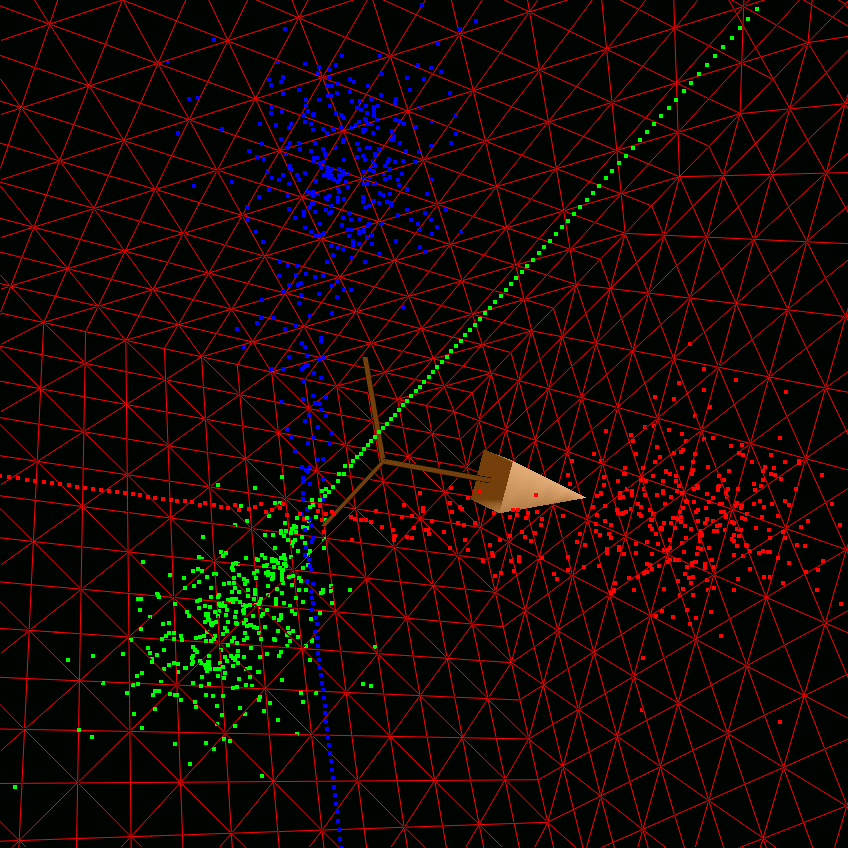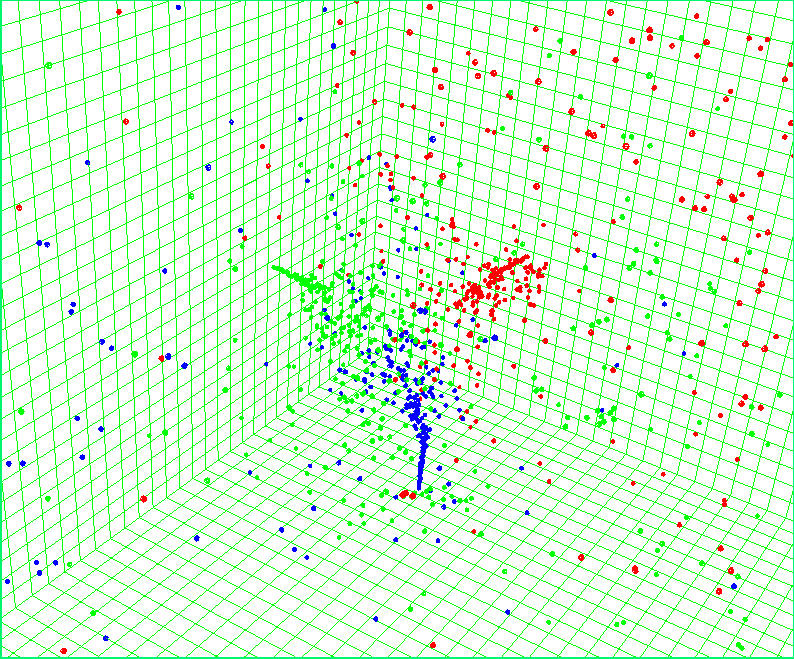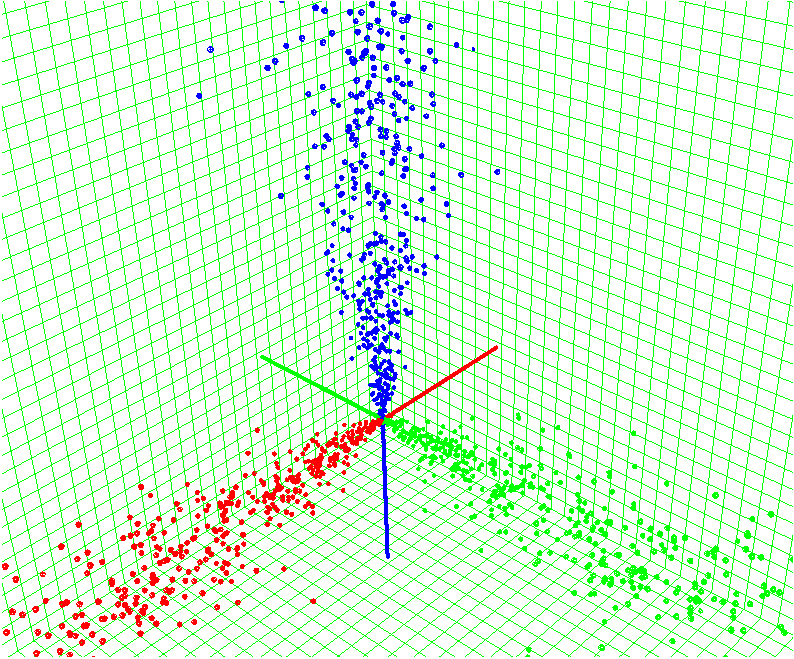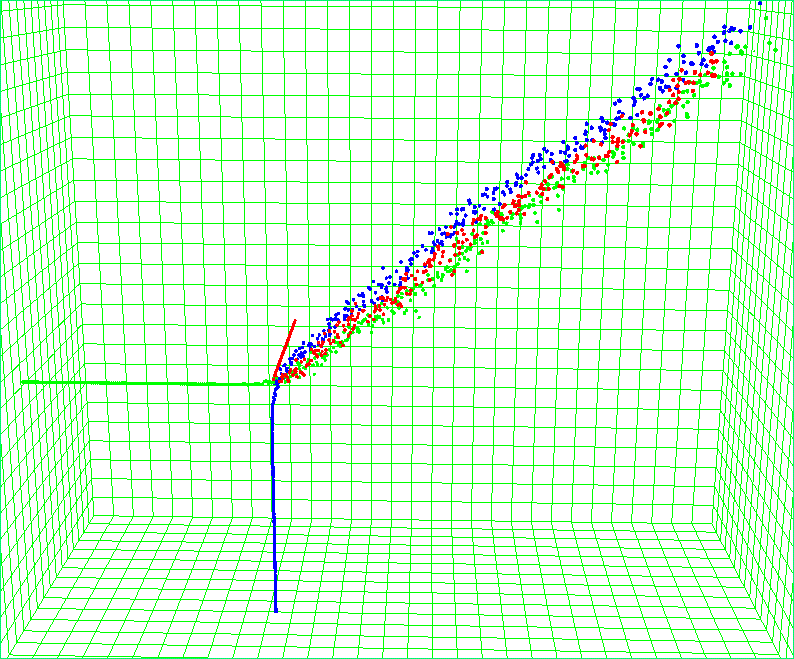# Particle Dynamics Modeling Using PII Scheme

#### Dynamics of impinging jets

Particle interaction mechanism is realized using a probabilistic implicit interaction scheme (PII) . The advantage of the scheme is that it provides a linear dependence of the execution time on the number of particles simulated.

PII is realized using a concept of virtual particles, which are the carriers of the interaction force, similar to the description of quantum field theory. Any real particle can convert a part of its energy, momentum, mass, etc. into a virtual particle, which in tern should return these quantities to a real particle. If there is no other real particle but the one that generated the virtual particle, the quantities borrowed by the virtual particle are returned back to the original particle. This would correspond to a free propagation without interactions. If on the other hand, there are other particles around, which could be the recepients of the quantities, an exchange of quantities may occur, which constitutes an interaction. In the end of every time-step all virtual particles should dissapear by dissipating all their quantitis to the real particles, thereby satisfying the conservation laws.

Introducing virtual particles makes the execution time of the code linear in the number of particles. This is due to the fact that instead of using a nested double-loop over the particles to analyse the interaction distances and forces, only two subsequent loops over real and virtual particles are needed to compute real-virtual and virtual-real interactions.

The interaction events between the real and virtual particles are governed by the interaction probabilities. In the current implementation the probabilities are represented by a one-parametric family of functions. A simple change in the single parameter may result in very different interaction mechanisms, which are shown below. In the simulation three particle jets were injected at right angles to each other from three faces of a cube, so that all will collide at a sinble impingement point.#### Hard-balls

[Hard-ball collision: three impinging jets]Animation

#### Deflection

[Deflection: three impinging jets]Animation

#### Soft-balls

[Soft-ball collision: three impinging jets]Animation#### References

 Smirnov, A. and Celik, I. "A Lagrangian Particle Dynamics Model with an Implicit Four-Way Coupling Scheme, The 2000 ASME International Mechanical Engineering Congress and Exposition. Fluids Engineering Division, vol.FED-253, Orlando, FL, Nov.5-11, 2000, pp.93-100, 2000.